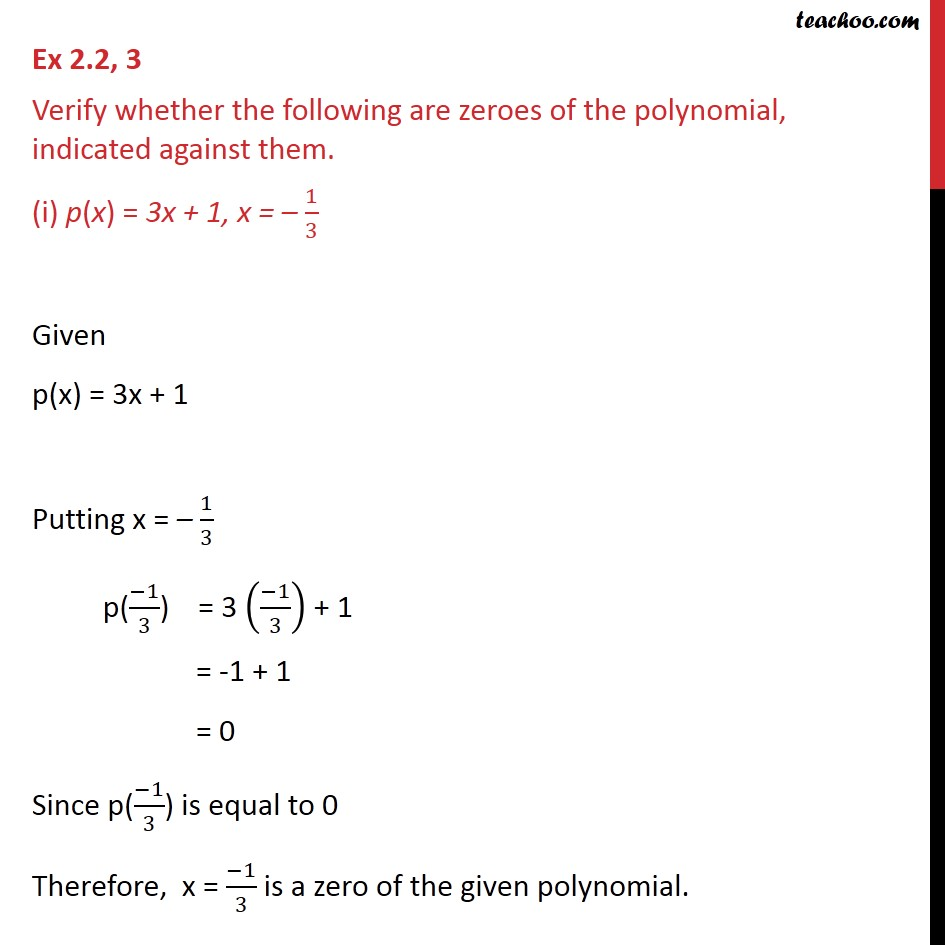1. Chapter 2 Class 9 Polynomials (Term 2)
2. Concept wise
3. Verifying Zeroes of a polynomial

Transcript

Ex 2.2, 3 Verify whether the following are zeroes of the polynomial, indicated against them. (i) p(x) = 3x + 1, x = – 1/3 Given p(x) = 3x + 1 Putting x = – 1/3 p((−1)/3) = 3 ((−1)/3) + 1 = -1 + 1 = 0 Since p((−1)/3) is equal to 0 Therefore, x = (−1)/3 is a zero of the given polynomial.